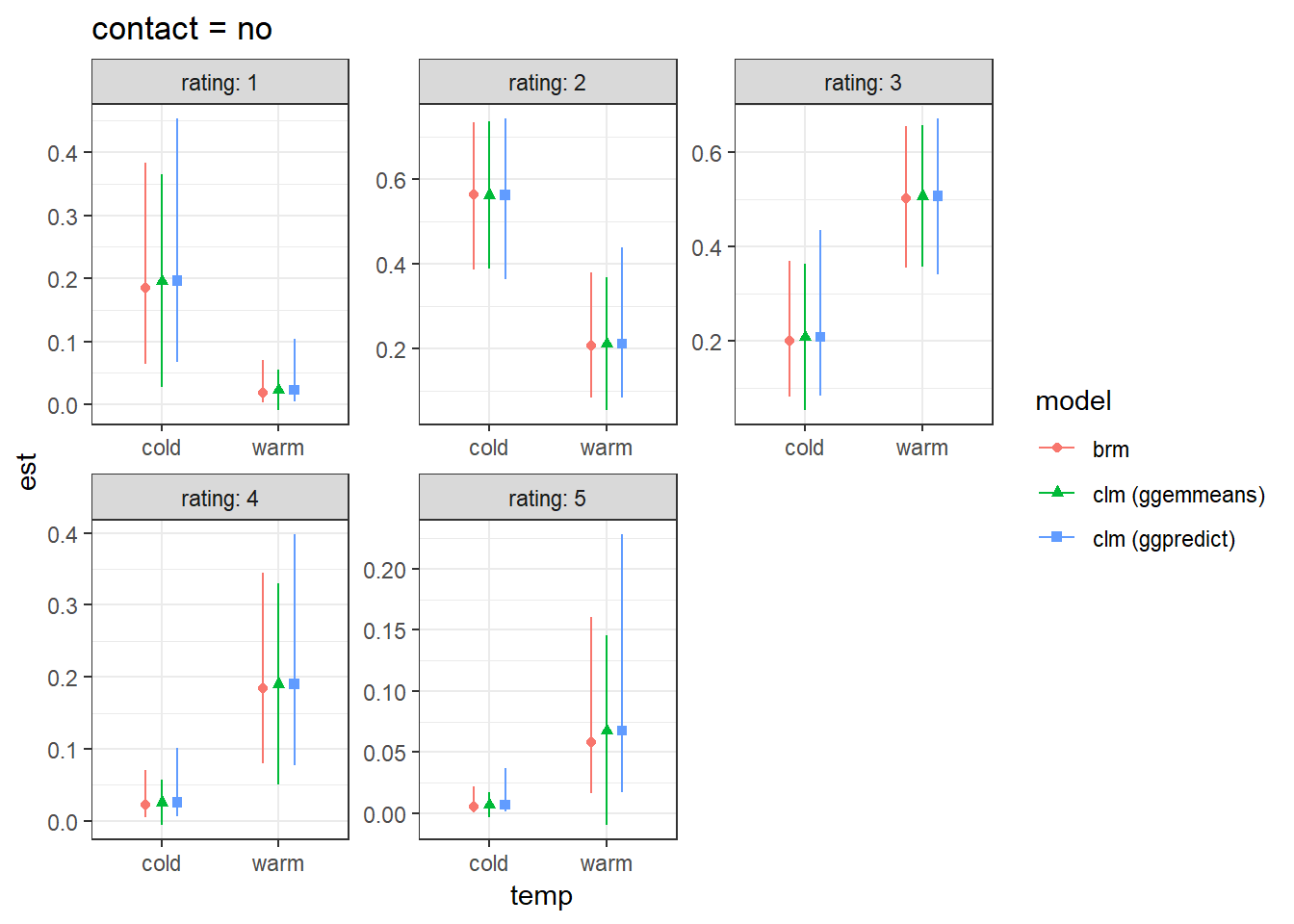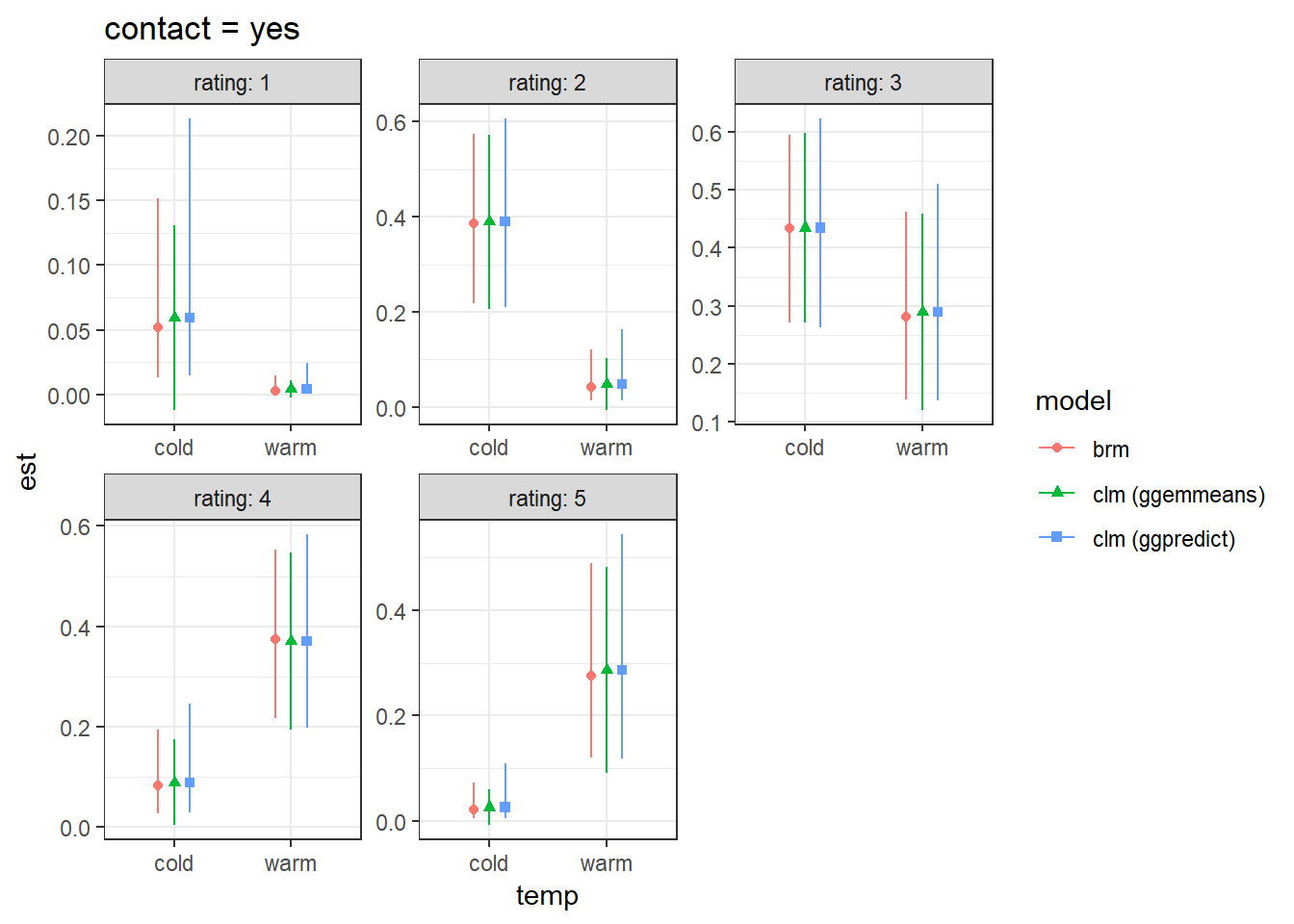This is a quick’n’dirty code for comparing CIs on predicted probabilities of ordinal logistic regression models, as supplement to http://rpubs.com/bbolker/ordpredprobCI

Predictions and CIs for clm’s from ggpredict() are based on predict(model, type = "prob", interval = se, level = ci, ...), while for ggemmeans() these are based on emmeans::emmeans(model, mode = "prob", ...) with confint() on the returned object from emmeans, to add confidence intervals.

library(sjmisc)
library(ggeffects)
library(ordinal)
library(brms)
library(MASS)  ## polr: *before* dplyr
library(dplyr)
library(tidyr)
library(tidybayes)
library(broom)
library(broom.mixed)
library(dotwhisker)
library(ggplot2);
theme_set(theme_bw())

m_clm <- clm(rating ~ temp * contact, data = wine)

m_brm <- brm(rating ~ temp * contact,
family =cumulative(threshold="flexible"),
data = wine,
refresh=0   ## quiet
)

pframe <- with(wine,expand.grid(temp=levels(temp), contact=levels(contact)))

pred_brm <- (tidybayes::fitted_draws(m_brm,pframe,category="rating")
%>% select(temp,contact,rating,.value)
%>% group_by(temp,contact,rating)
%>% summarise(lo=quantile(.value,0.025),
est=median(.value),
hi=quantile(.value,0.975))
%>% ungroup
)

pred_all <- pred_brm
pred_all$model <- "brm" pr <- ggpredict(m_clm, c("temp", "contact"), x.as.factor = T) pr <- as.data.frame(var_rename( pr, group = "contact", conf.high = "hi", conf.low = "lo", response.level = "rating", predicted = "est") ) colnames(pr) <- "temp" pr$model <- "clm (ggpredict)"

pr2 <- ggemmeans(m_clm, c("temp", "contact"), x.as.factor = T)
pr2 <- as.data.frame(var_rename(
pr2, group = "contact", conf.high = "hi", conf.low = "lo",
response.level = "rating", predicted = "est")
)

colnames(pr2) <- "temp"
pr2\$model <- "clm (ggemmeans)"

pr_final <- merge_df(pred_all, pr, pr2)

gg0 <- ggplot(dplyr::filter(pr_final, model != "clm", contact == "no") ,aes(temp, est, colour = model)) +
geom_point(aes(shape = model), position = position_dodge(.4)) +
geom_errorbar(aes(ymin = lo, ymax = hi), width = 0, position = position_dodge(.4))
gg0 + facet_wrap(~rating, labeller=label_both, drop = T, scales = "free") +
labs(title = "contact = no")gg0 <- ggplot(dplyr::filter(pr_final, model != "clm", contact == "yes") ,aes(temp, est, colour = model)) +
geom_point(aes(shape = model), position = position_dodge(.4)) +
geom_errorbar(aes(ymin = lo, ymax = hi), width = 0, position = position_dodge(.4))
gg0 + facet_wrap(~rating, labeller=label_both, drop = T, scales = "free") +
labs(title = "contact = yes")